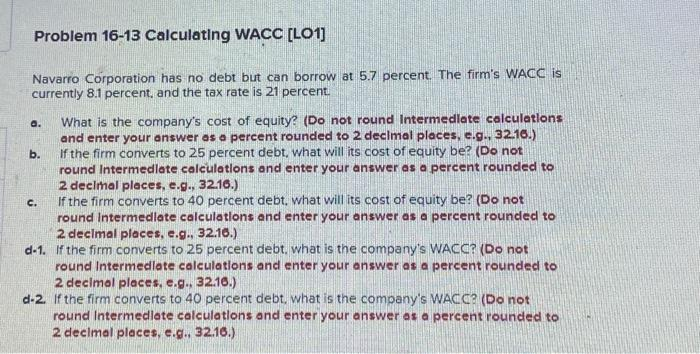Home / Expert Answers / Finance / problem-16-13-calculating-wacc-lo1-navarro-corporation-has-no-debt-but-can-borrow-at-5-7-percent-pa780

# (Solved): Problem 16-13 Calculating WACC [LO1] Navarro Corporation has no debt but can borrow at 5.7 percent. ...Problem 16-13 Calculating WACC [LO1] Navarro Corporation has no debt but can borrow at 5.7 percent. The firm's WACC is currently 8.1 percent, and the tax rate is 21 percent. a. What is the company's cost of equity? (Do not round Intermedlate colculations and enter your answer os o percent rounded to 2 declmal places, e.g., 32.16.) b. If the firm converts to 25 percent debt, what will its cost of equity be? (Do not round Intermedlate calculations and enter your answer as a percent rounded to 2 decimal ploces, e.g., 32.16.) c. If the firm converts to 40 percent debt, what will its cost of equity be? (Do not round Intermedlote calculations and enter your answer as a percent rounded to 2 decimal places, e.g., 32.16.) d-1. If the firm converts to 25 percent debt, what is the company's WACC? (Do not round Intermedlate calculations and enter your answer as a percent rounded to 2 decimol places, e.g., 32.16.) d-2. If the firm converts to 40 percent debt, what is the company's WACC? (Do not round Intermedlote calculations and enter your answer os a percent rouncled to 2 decimal places, e.g., 32.16.)

We have an Answer from Expert